# Science 10 Chapter B 2 ENERGY TRANSFORMATIONS B

• Slides: 57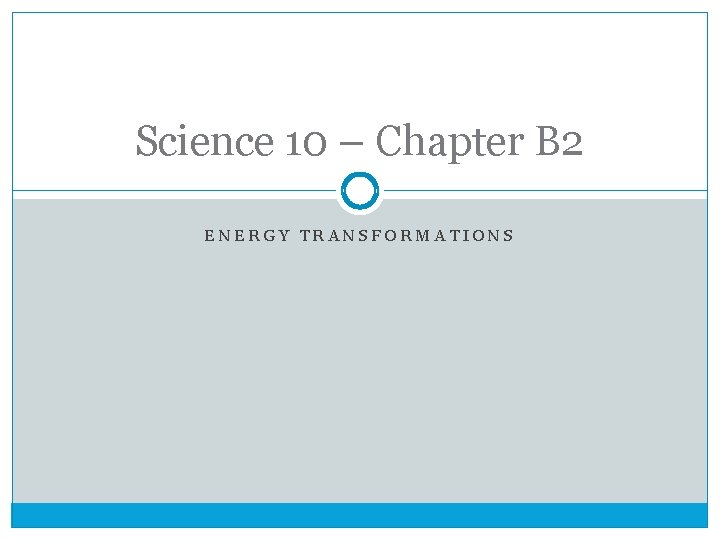Science 10 – Chapter B 2 ENERGY TRANSFORMATIONS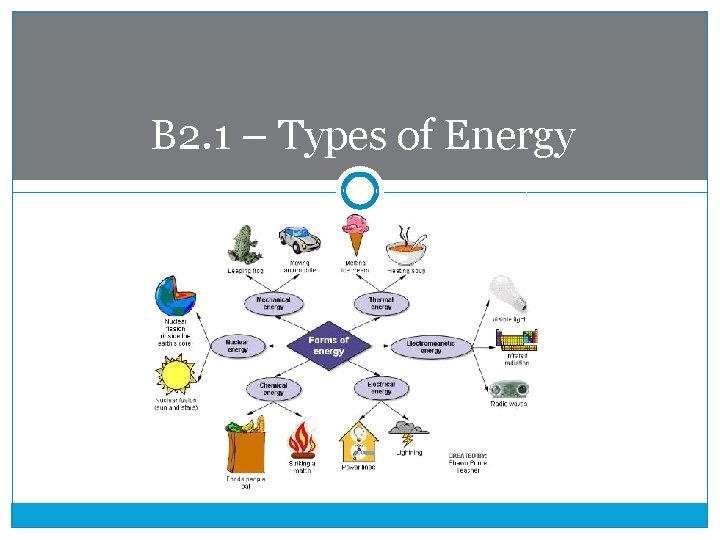B 2. 1 – Types of EnergyTypes of Energy �There are many types of energy, and often in nature and in mechanical devices, we see a transformation of energy from one type to another �As we will see, in any transformation, energy is never created or destroyed �Energy can be transferred from one object to another or transformed from one type to another �While there are many types of energy, many forms can be classified as either potential or kinetic energy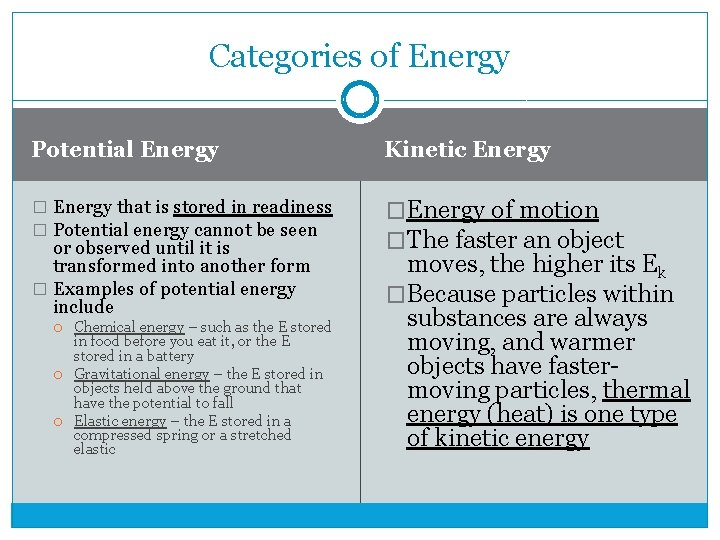Categories of Energy Potential Energy Kinetic Energy � Energy that is stored in readiness � Potential energy cannot be seen �Energy of motion �The faster an object or observed until it is transformed into another form � Examples of potential energy include Chemical energy – such as the E stored in food before you eat it, or the E stored in a battery Gravitational energy – the E stored in objects held above the ground that have the potential to fall Elastic energy – the E stored in a compressed spring or a stretched elastic moves, the higher its Ek �Because particles within substances are always moving, and warmer objects have fastermoving particles, thermal energy (heat) is one type of kinetic energyNuclear energy �Another type of potential energy, nuclear energy is energy stored in the nucleus of an atom �It can be released during a nuclear reaction, such as: Radioactive decay Nuclear fusion – when the nuclei of two atoms fuse to make one larger atom � This is the type of reaction that occurs in the sun to produce solar energy Nuclear fission – when one atom breaks apart to form two or more smaller atoms � This is the type of reaction that occurs in a nuclear power plant and in a nuclear bombEnergy conversions �An energy conversion refers to the transformation of energy from one form to another �In each example below, a device converts one type of energy input into a different type of energy output A flashlight converts chemical potential energy into electromagnetic (light) energy A windmill converts kinetic energy into electrical energy A car engine converts chemical potential energy into kinetic energy A solar panel converts electromagnetic (solar) energy into electrical energyVolta Pile – the first battery �one notable energy conversion was a device invented by Alessandro Volta called the Volta pile �the Volta Pile converted the chemical potential energy of different metals into electrical energy and was, therefore, the first batteryJoule’s experiments �James Prescott Joule performed two experiments to demonstrate energy transformations: Experiment #1: showed that a falling object (that had potential energy) can create heat (thermal energy) Experiment #2: showed that a moving object (that had kinetic energy) can create heat Because of his work on energy, the unit we use to quantify it is called the joule �Joule’s work came at an important time – working at the start of the Industrial Revolution, people were very interested in designing machines that could perform useful work – that is, transform one type of energy into mechanical energy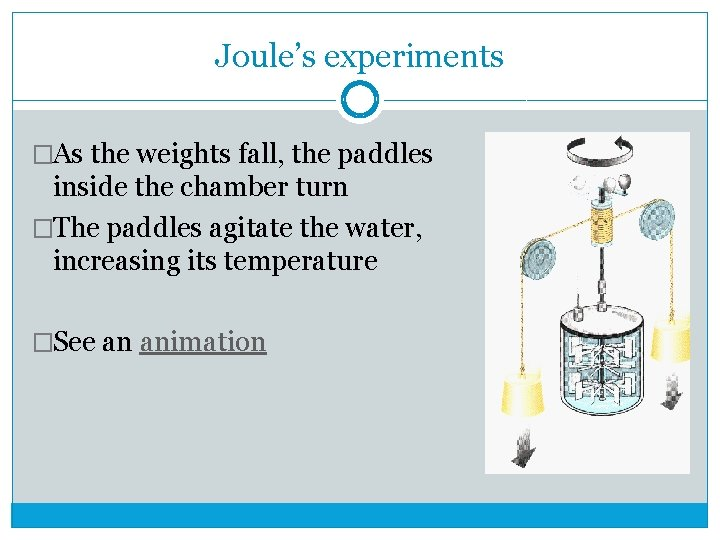Joule’s experiments �As the weights fall, the paddles inside the chamber turn �The paddles agitate the water, increasing its temperature �See an animation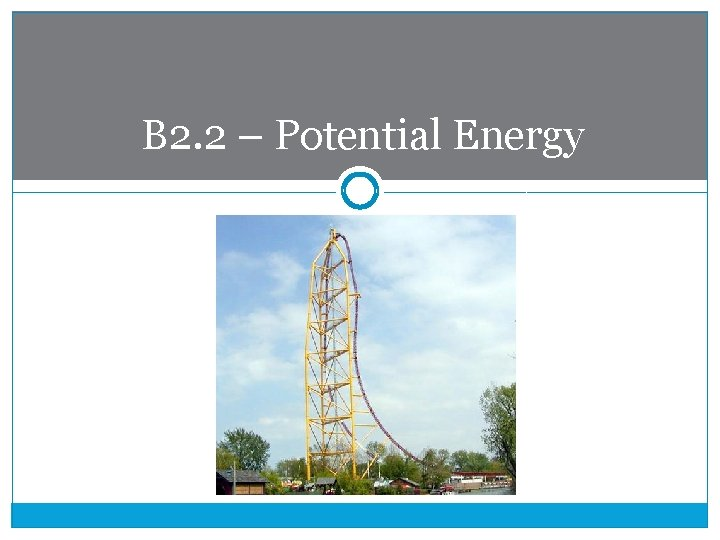B 2. 2 – Potential EnergyWhat is potential energy? �Recall, potential energy is energy stored in readiness �It can only be observed once it is transformed into some other type of energy E. g. Gravity cannot be observed until you allow an object to fall – Ep(grav) is converted into Ek E. g. Chemical energy in a fuel cannot be observed, but once the fuel burns in your gas tank the car moves – Ep(chem) is converted into EmechanicalGravitational potential energy �An object has Ep(grav) if it has the potential to fall That is, if its position is above the Earth’s surface �The greater the mass of the object, or the higher it is, the greater its Ep(grav) �The formula for gravitational potential energy is: Ep(grav) = mgh, where � m = the mass of the object, in kg � g = the acceleration of the object due to gravity = 9. 81 m/s 2 � h = the height of the object, in m Like all types of energy, Ep(grav) is measured in joules (J)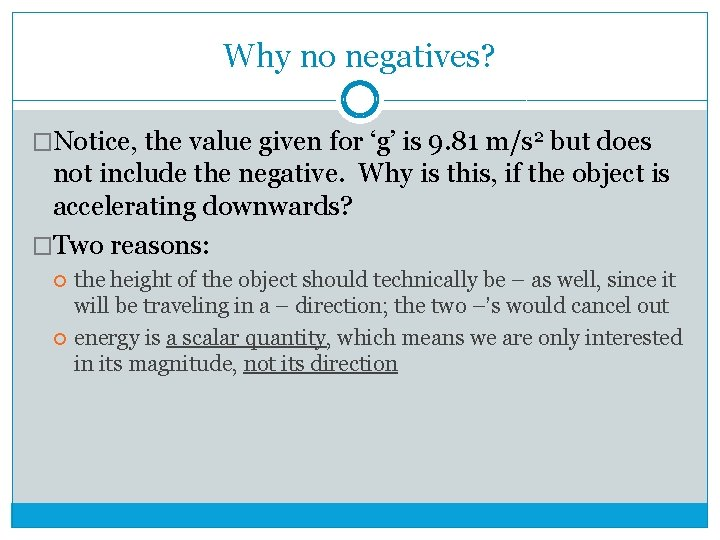Why no negatives? �Notice, the value given for ‘g’ is 9. 81 m/s 2 but does not include the negative. Why is this, if the object is accelerating downwards? �Two reasons: the height of the object should technically be – as well, since it will be traveling in a – direction; the two –’s would cancel out energy is a scalar quantity, which means we are only interested in its magnitude, not its directionExample: �A 80 kg diver handstands on a 10 m diving platform. Before diving, how much gravitational potential energy does the diver have? �Ep = mgh = (80 kg)(9. 81 m/s 2)(10 m) = 7848 J = 7. 8 x 103 JWeight vs. mass �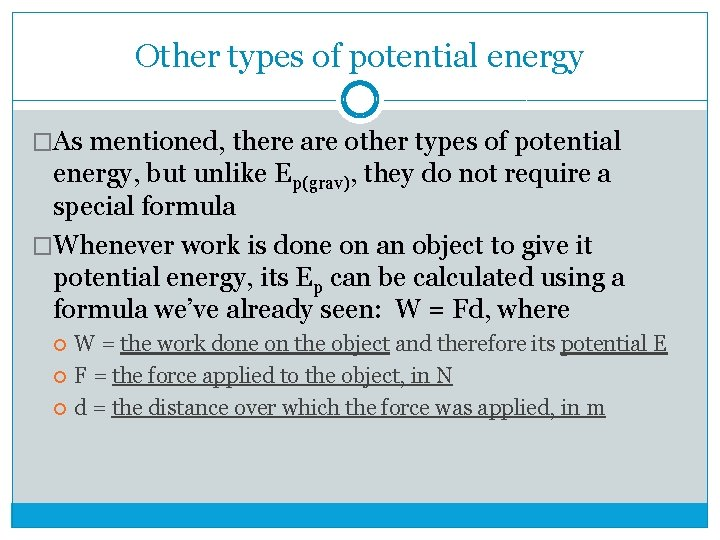Other types of potential energy �As mentioned, there are other types of potential energy, but unlike Ep(grav), they do not require a special formula �Whenever work is done on an object to give it potential energy, its Ep can be calculated using a formula we’ve already seen: W = Fd, where W = the work done on the object and therefore its potential E F = the force applied to the object, in N d = the distance over which the force was applied, in m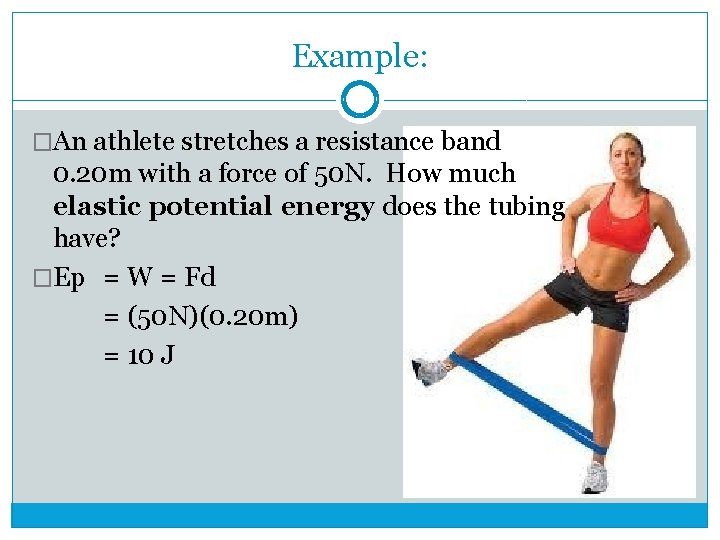Example: �An athlete stretches a resistance band 0. 20 m with a force of 50 N. How much elastic potential energy does the tubing have? �Ep = W = Fd = (50 N)(0. 20 m) = 10 J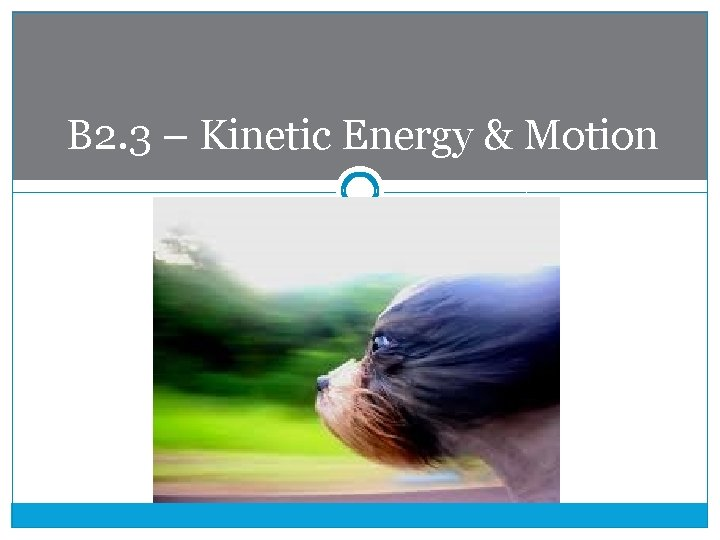B 2. 3 – Kinetic Energy & Motion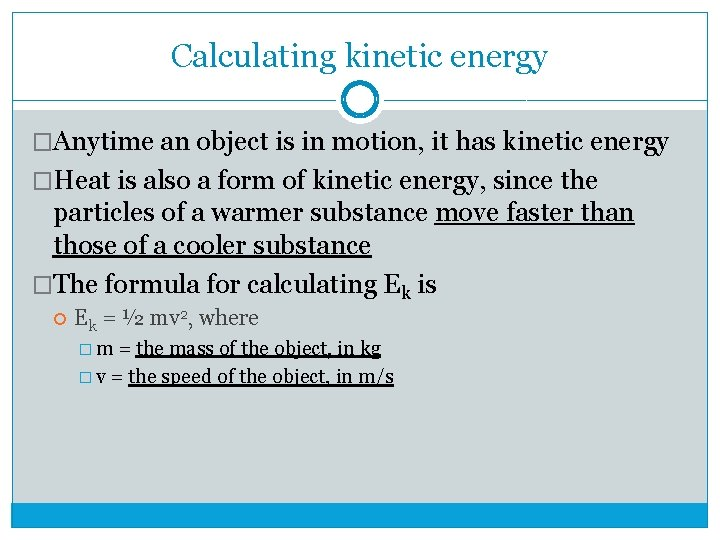Calculating kinetic energy �Anytime an object is in motion, it has kinetic energy �Heat is also a form of kinetic energy, since the particles of a warmer substance move faster than those of a cooler substance �The formula for calculating Ek is Ek = ½ mv 2, where � m = the mass of the object, in kg � v = the speed of the object, in m/s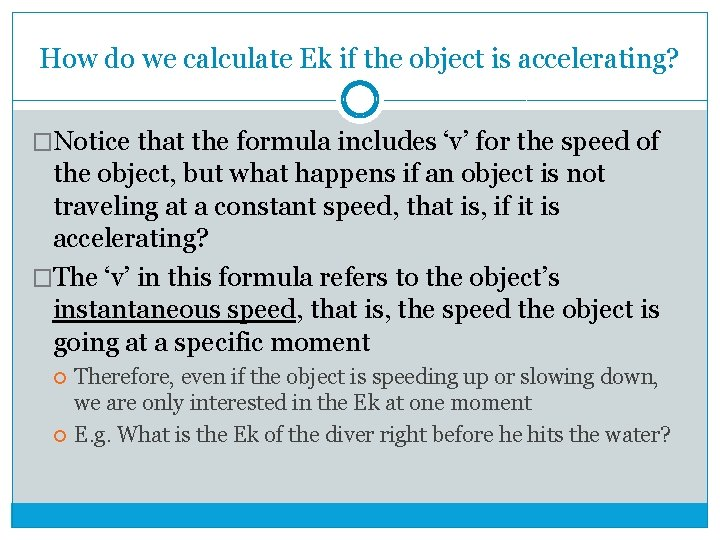How do we calculate Ek if the object is accelerating? �Notice that the formula includes ‘v’ for the speed of the object, but what happens if an object is not traveling at a constant speed, that is, if it is accelerating? �The ‘v’ in this formula refers to the object’s instantaneous speed, that is, the speed the object is going at a specific moment Therefore, even if the object is speeding up or slowing down, we are only interested in the Ek at one moment E. g. What is the Ek of the diver right before he hits the water?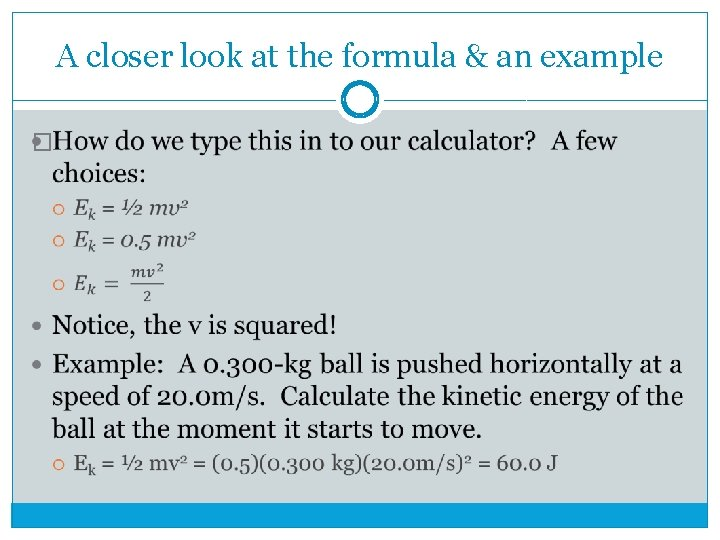A closer look at the formula & an example �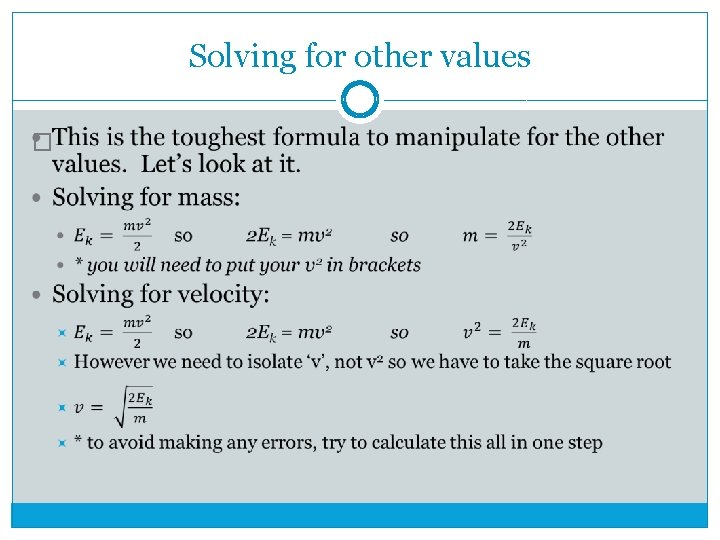Solving for other values �Example �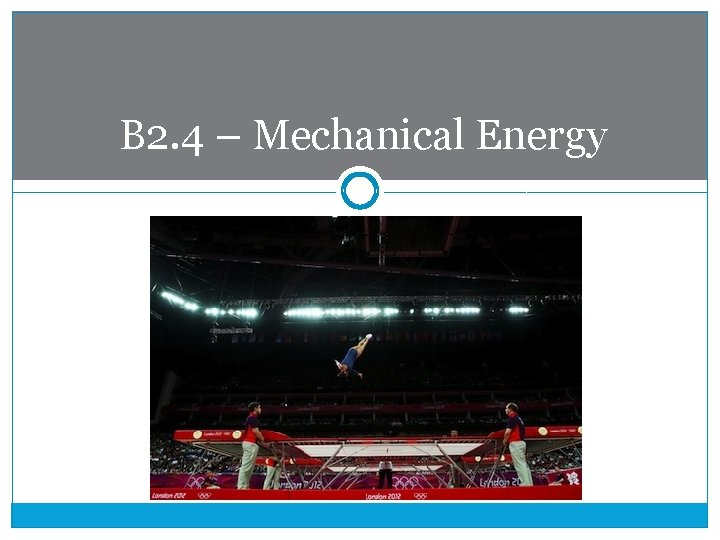B 2. 4 – Mechanical Energy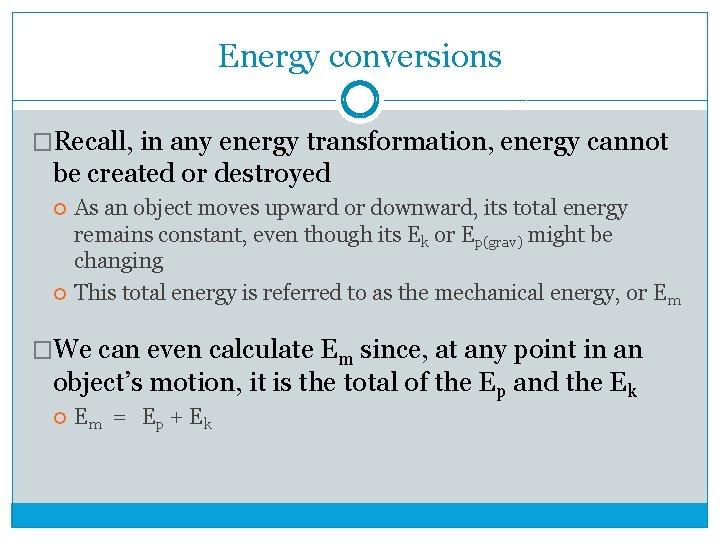Energy conversions �Recall, in any energy transformation, energy cannot be created or destroyed As an object moves upward or downward, its total energy remains constant, even though its Ek or Ep(grav) might be changing This total energy is referred to as the mechanical energy, or Em �We can even calculate Em since, at any point in an object’s motion, it is the total of the Ep and the Ek Em = Ep + Ek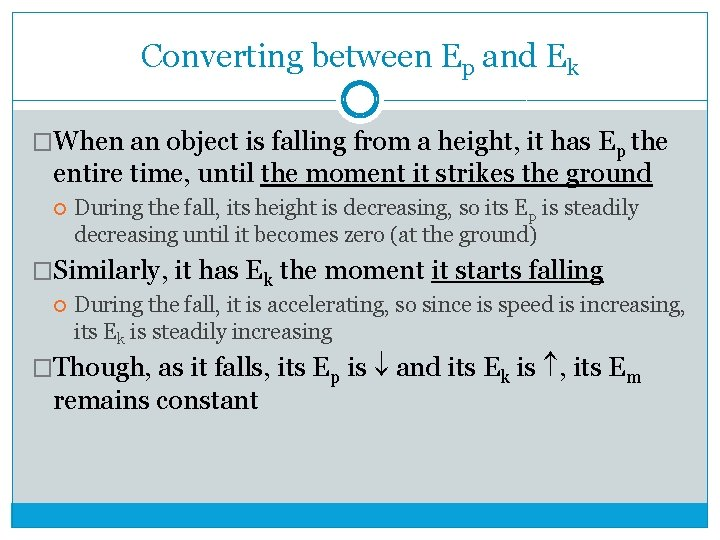Converting between Ep and Ek �When an object is falling from a height, it has Ep the entire time, until the moment it strikes the ground During the fall, its height is decreasing, so its Ep is steadily decreasing until it becomes zero (at the ground) �Similarly, it has Ek the moment it starts falling During the fall, it is accelerating, so since is speed is increasing, its Ek is steadily increasing �Though, as it falls, its Ep is and its Ek is , its Em remains constant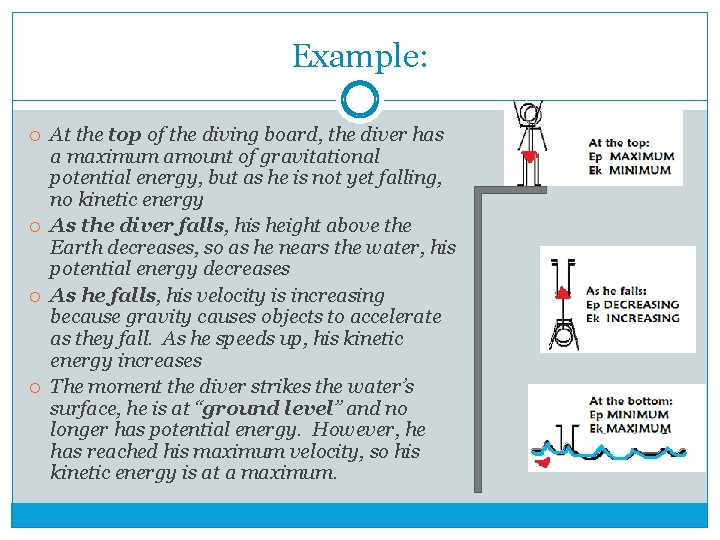Example: At the top of the diving board, the diver has a maximum amount of gravitational potential energy, but as he is not yet falling, no kinetic energy As the diver falls, his height above the Earth decreases, so as he nears the water, his potential energy decreases As he falls, his velocity is increasing because gravity causes objects to accelerate as they fall. As he speeds up, his kinetic energy increases The moment the diver strikes the water’s surface, he is at “ground level” and no longer has potential energy. However, he has reached his maximum velocity, so his kinetic energy is at a maximum.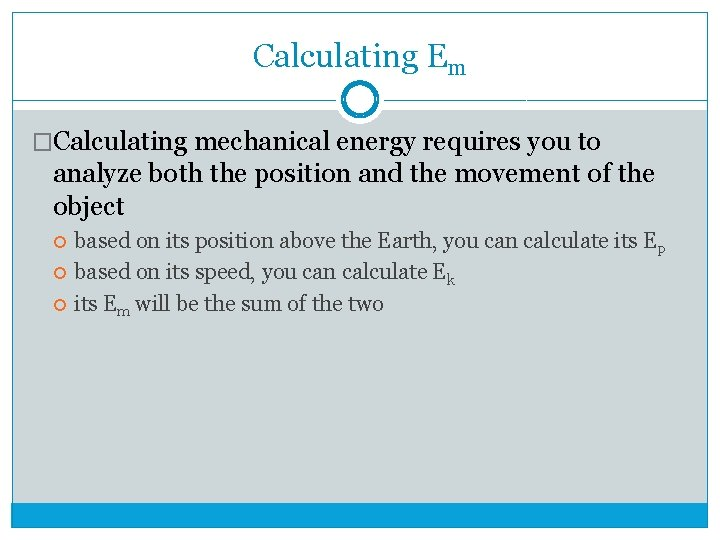Calculating Em �Calculating mechanical energy requires you to analyze both the position and the movement of the object based on its position above the Earth, you can calculate its Ep based on its speed, you can calculate Ek its Em will be the sum of the twoExample: �A roller coaster and its passengers have a combined mass of 1000 kg. Halfway down one slope, the coaster is 20. 0 m off the ground and traveling at 5. 00 m/s. What is the mechanical energy of the coaster at that point? Em = Ep + Ek = mgh + ½ mv 2 = (1000 kg)(9. 81 m/s 2)(20. 0 m) + ½ (1000 kg)(5. 00 m/s)2 = 196 200 J + 12 500 J = 208 700 J = 2. 09 x 105 J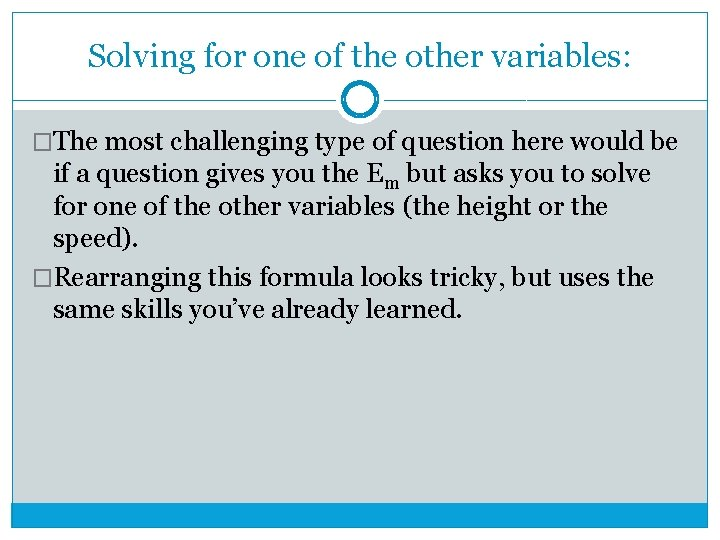Solving for one of the other variables: �The most challenging type of question here would be if a question gives you the Em but asks you to solve for one of the other variables (the height or the speed). �Rearranging this formula looks tricky, but uses the same skills you’ve already learned.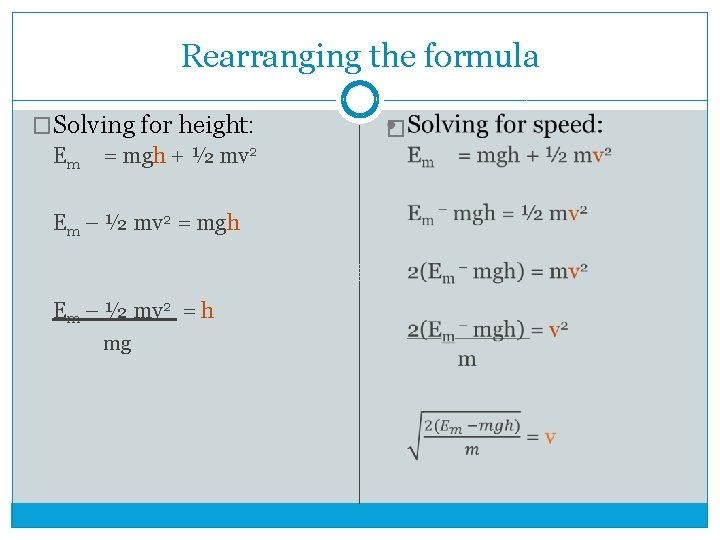Rearranging the formula �Solving for height: Em = mgh + ½ mv 2 Em – ½ mv 2 = mgh Em – ½ mv 2 = h mg �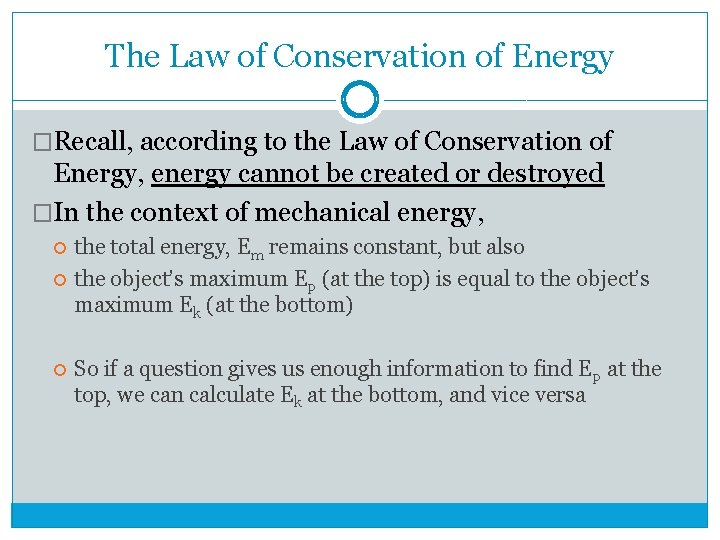The Law of Conservation of Energy �Recall, according to the Law of Conservation of Energy, energy cannot be created or destroyed �In the context of mechanical energy, the total energy, Em remains constant, but also the object’s maximum Ep (at the top) is equal to the object’s maximum Ek (at the bottom) So if a question gives us enough information to find Ep at the top, we can calculate Ek at the bottom, and vice versa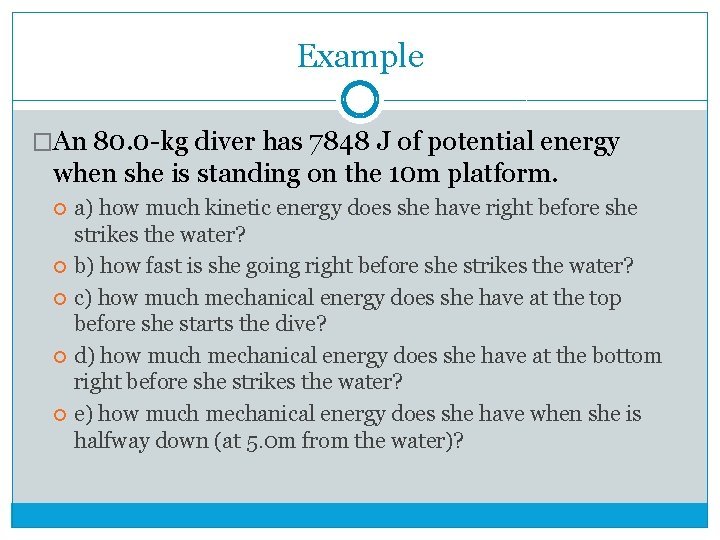Example �An 80. 0 -kg diver has 7848 J of potential energy when she is standing on the 10 m platform. a) how much kinetic energy does she have right before she strikes the water? b) how fast is she going right before she strikes the water? c) how much mechanical energy does she have at the top before she starts the dive? d) how much mechanical energy does she have at the bottom right before she strikes the water? e) how much mechanical energy does she have when she is halfway down (at 5. 0 m from the water)?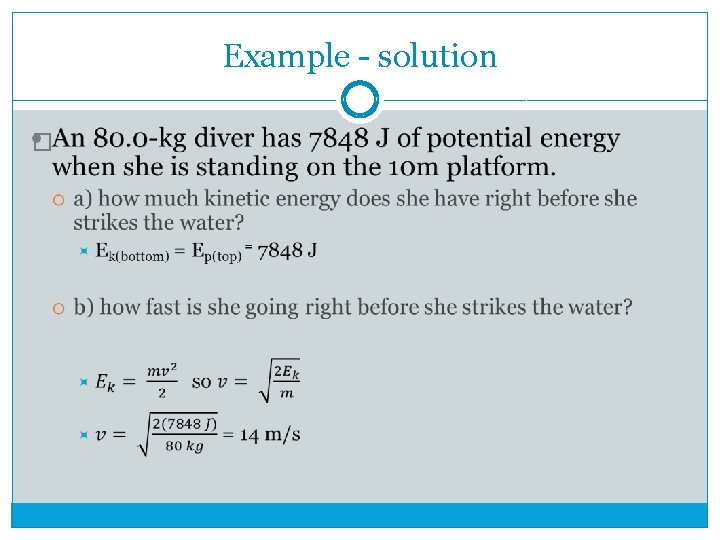Example - solution �Example - solution �An 80. 0 -kg diver has 7848 J of potential energy when she is standing on the 10 m platform. c) how much mechanical energy does she have at the top before she starts the dive? � Em = Ep + Ek before she starts to move, her Ek is zero � Em = 7848 J + 0 J = 7848 J d) how much mechanical energy does she have at the bottom right before she strikes the water? � Em = Ep + Ek as she is at her lowest height, her Ep is zero � Em = 0 J + 7848 J = 7848 J e) how much mechanical energy does she have when she is halfway down (at 5. 0 m from the water)? � At any point, her Em remains constant, at 7848 J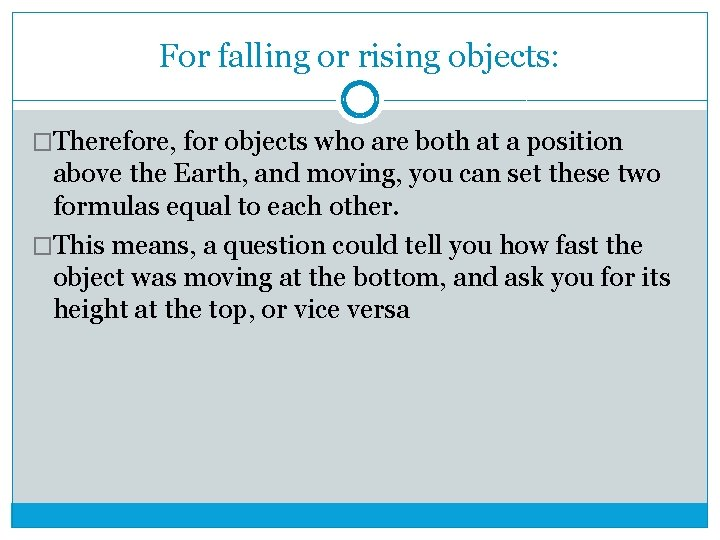For falling or rising objects: �Therefore, for objects who are both at a position above the Earth, and moving, you can set these two formulas equal to each other. �This means, a question could tell you how fast the object was moving at the bottom, and ask you for its height at the top, or vice versa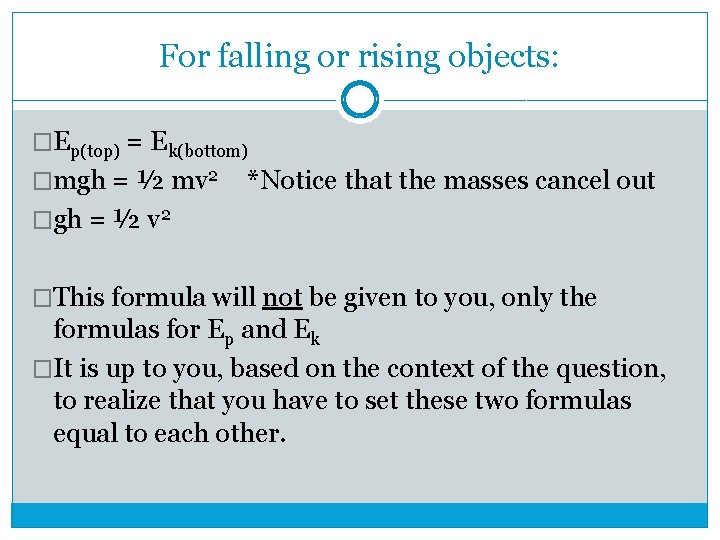For falling or rising objects: �Ep(top) = Ek(bottom) �mgh = ½ mv 2 *Notice that the masses cancel out �gh = ½ v 2 �This formula will not be given to you, only the formulas for Ep and Ek �It is up to you, based on the context of the question, to realize that you have to set these two formulas equal to each other.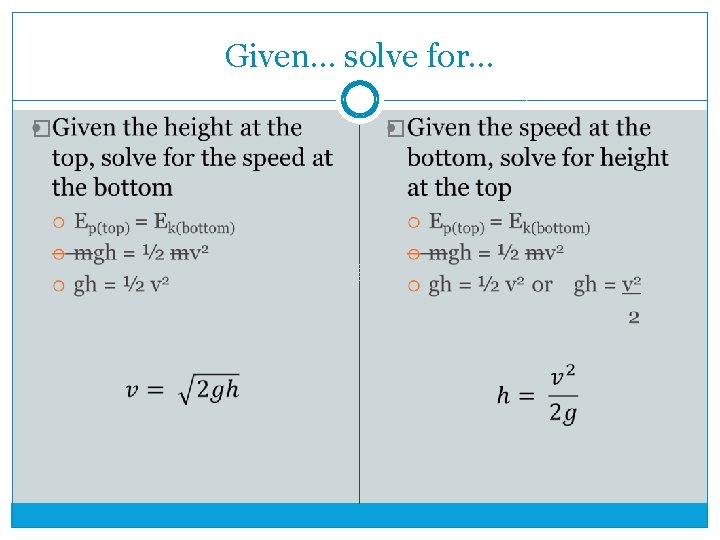Given… solve for… � �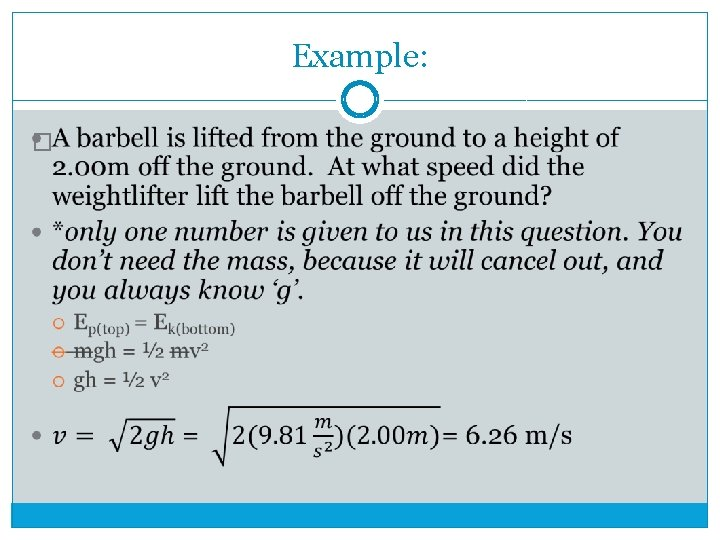Example: �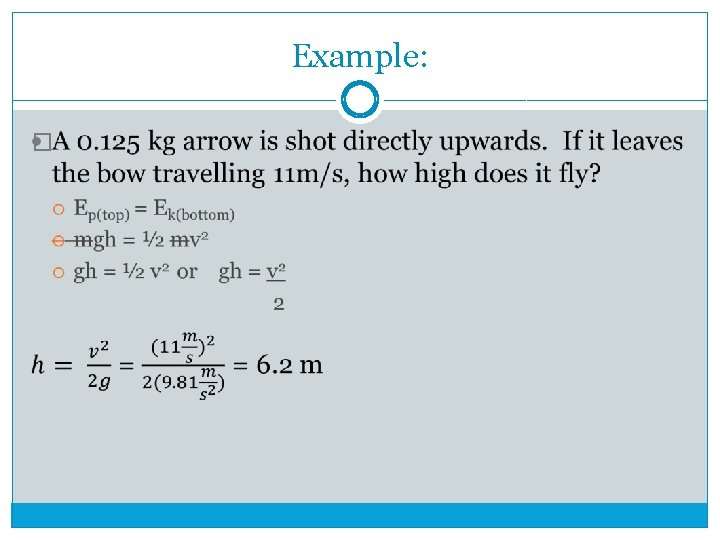Example: �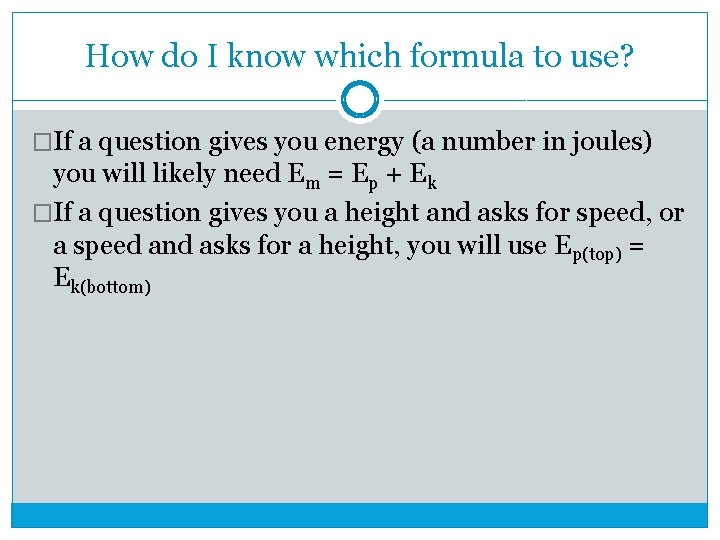How do I know which formula to use? �If a question gives you energy (a number in joules) you will likely need Em = Ep + Ek �If a question gives you a height and asks for speed, or a speed and asks for a height, you will use Ep(top) = Ek(bottom)One more example �Though not an example of an object rising or falling, a pendulum is another great example of a constant transformation between Ek and Ep.Pendulums �another example of a conversion between Ep and Ek at position A, the pendulum is not moving, but is at a maximum height maximum Ep, minimum Ek at position B, the pendulum is moving at a maximum speed, but is the closest to the ground minimum Ep, maximum Ek at position C, the pendulum is at the same position as position A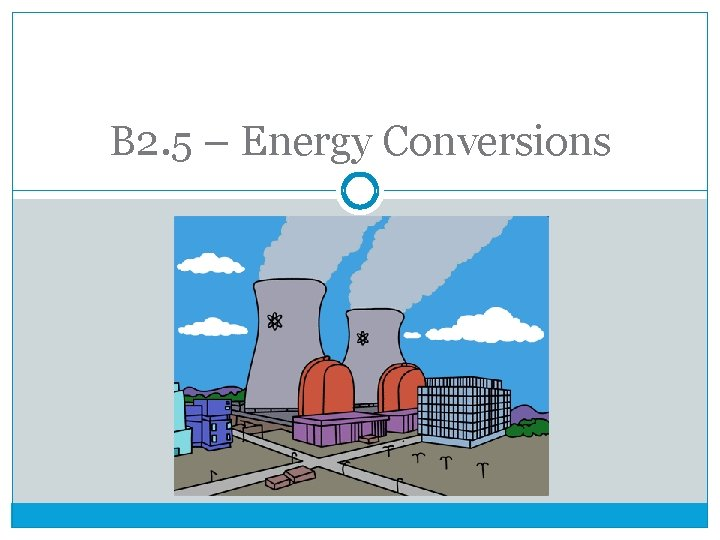B 2. 5 – Energy ConversionsEvidence of Energy Conversions �as we’ve seen, an energy conversion is the transformation of energy from one type to another �different results can occur as a result: motion – an object can move a change in position – an object can rise or fall a change in shape – an elastic could stretch, or a spring compress a change in temperature – an object could heat upEnergy conversions in nature �Two major energy conversions occur in nature, and will be addressed in the Biology Unit: photosynthesis is the conversion of solar energy into chemical potential energy and is performed by plants cellular respiration is the conversion of chemical potential energy into useful energy, e. g. energy of motion, thermal energy, etc. and is performed by all living organisms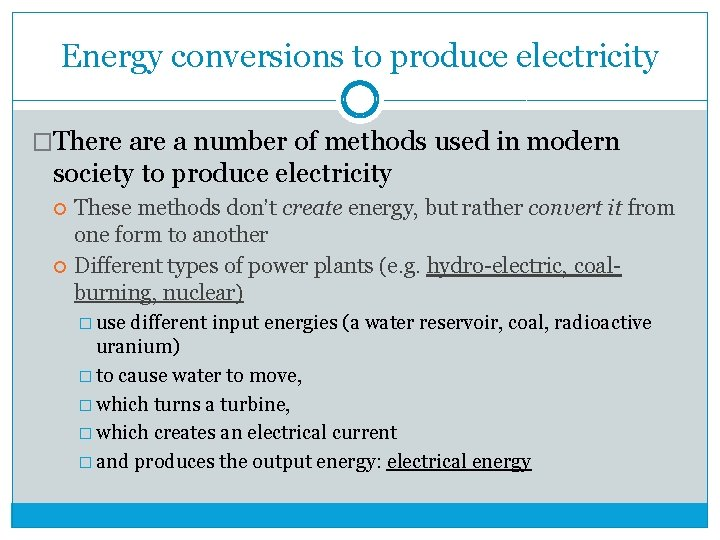Energy conversions to produce electricity �There a number of methods used in modern society to produce electricity These methods don’t create energy, but rather convert it from one form to another Different types of power plants (e. g. hydro-electric, coalburning, nuclear) � use different input energies (a water reservoir, coal, radioactive uranium) � to cause water to move, � which turns a turbine, � which creates an electrical current � and produces the output energy: electrical energyHydro-electric power stations �Uses the gravitational potential energy of water that is in a position above the power plant �when the water falls, it gains kinetic energy �this fast-moving water makes the turbine spin and produces electricity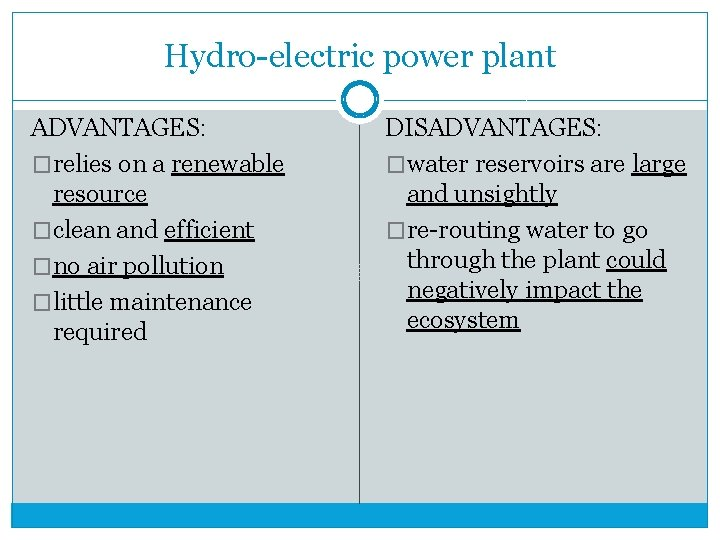Hydro-electric power plant ADVANTAGES: �relies on a renewable resource �clean and efficient �no air pollution �little maintenance required DISADVANTAGES: �water reservoirs are large and unsightly �re-routing water to go through the plant could negatively impact the ecosystemCoal-burning power plants �also known as thermoelectric power plants �convert chemical energy stored in fossil fuels into electric energy �when the fuel is burned, the chemical energy is released �this is transformed into thermal energy which is used to heat steam �this fast-moving steam makes the turbine turn, producing electricity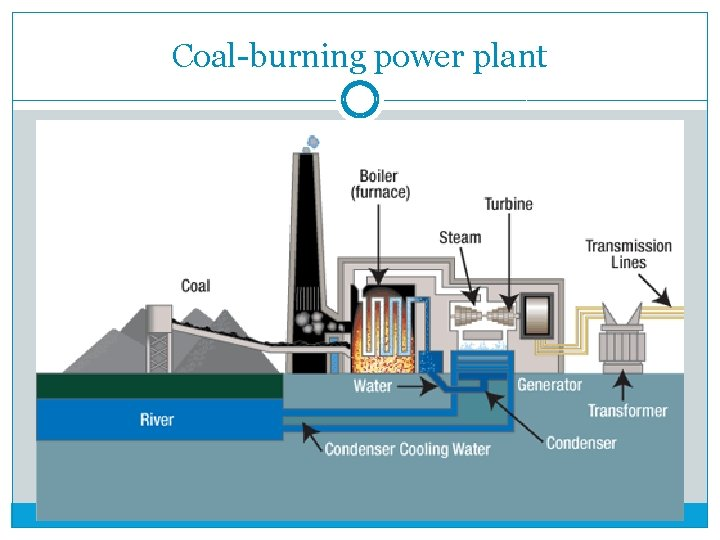Coal-burning power plant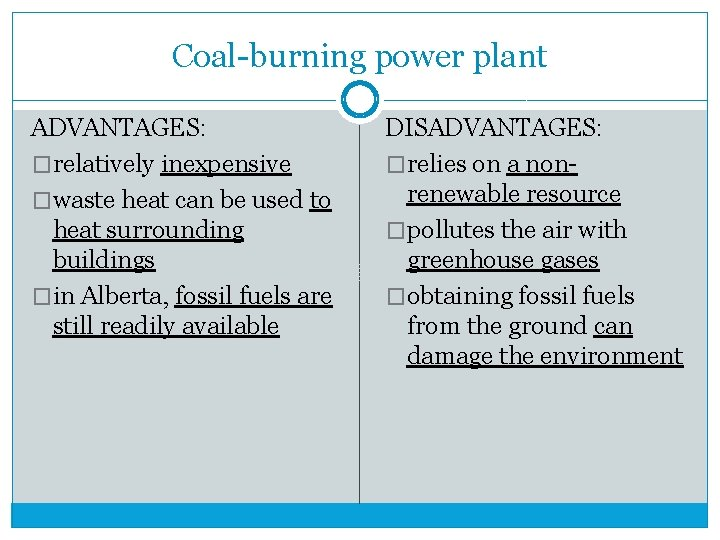Coal-burning power plant ADVANTAGES: �relatively inexpensive �waste heat can be used to heat surrounding buildings �in Alberta, fossil fuels are still readily available DISADVANTAGES: �relies on a nonrenewable resource �pollutes the air with greenhouse gases �obtaining fossil fuels from the ground can damage the environmentNuclear power plant �atoms of uranium are split by nuclear fission �splitting atoms releases a tremendous amount of thermal energy �this thermal energy is used to make steam, which turns a turbine, and again, produces electricityNuclear power plantNuclear power plant ADVANTAGES: �do not produce harmful greenhouse gases �very efficient: a small amount of uranium produces an enormous amount of electricity DISADVANTAGES: �nuclear waste is dangerous to any living thing exposed to it for 100 s of years �facilities for storing nuclear waste are expensiveSolar cells �An alternative to a conventional power plant, solar cells produce electrical energy directly from solar energy without the need of a rotating turbineHydrogen fuel cells �A hydrogen fuel cell is another alternative to fossil fuels to produce electricity �It uses the formation reaction of water, H 2(g) + O 2(g) H 2 O(l) to produce electricity �Because the product is water, fuel cells do not produce harmful carbon compounds �Unfortunately, due to lack in availability of pure hydrogen, a high risk of explosion, and the large size of these fuel cells, they are not yet a viable alternative to gasoline engines